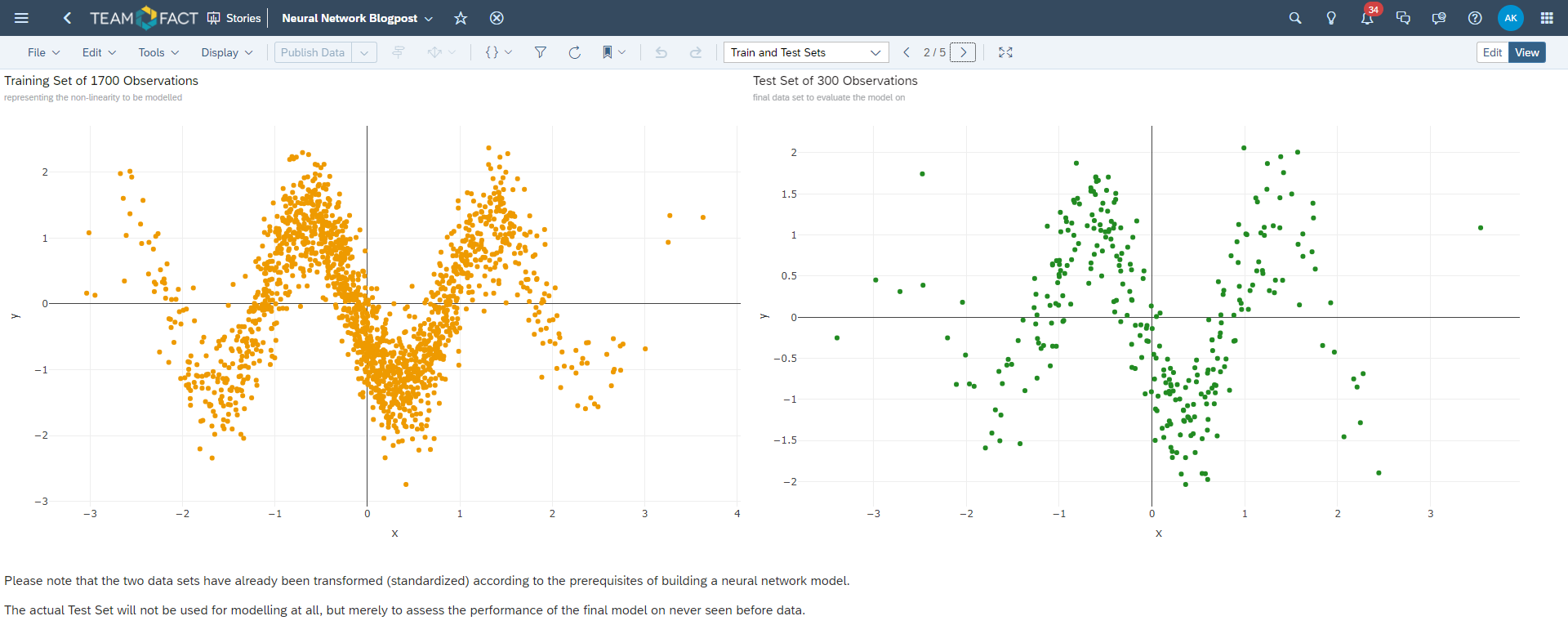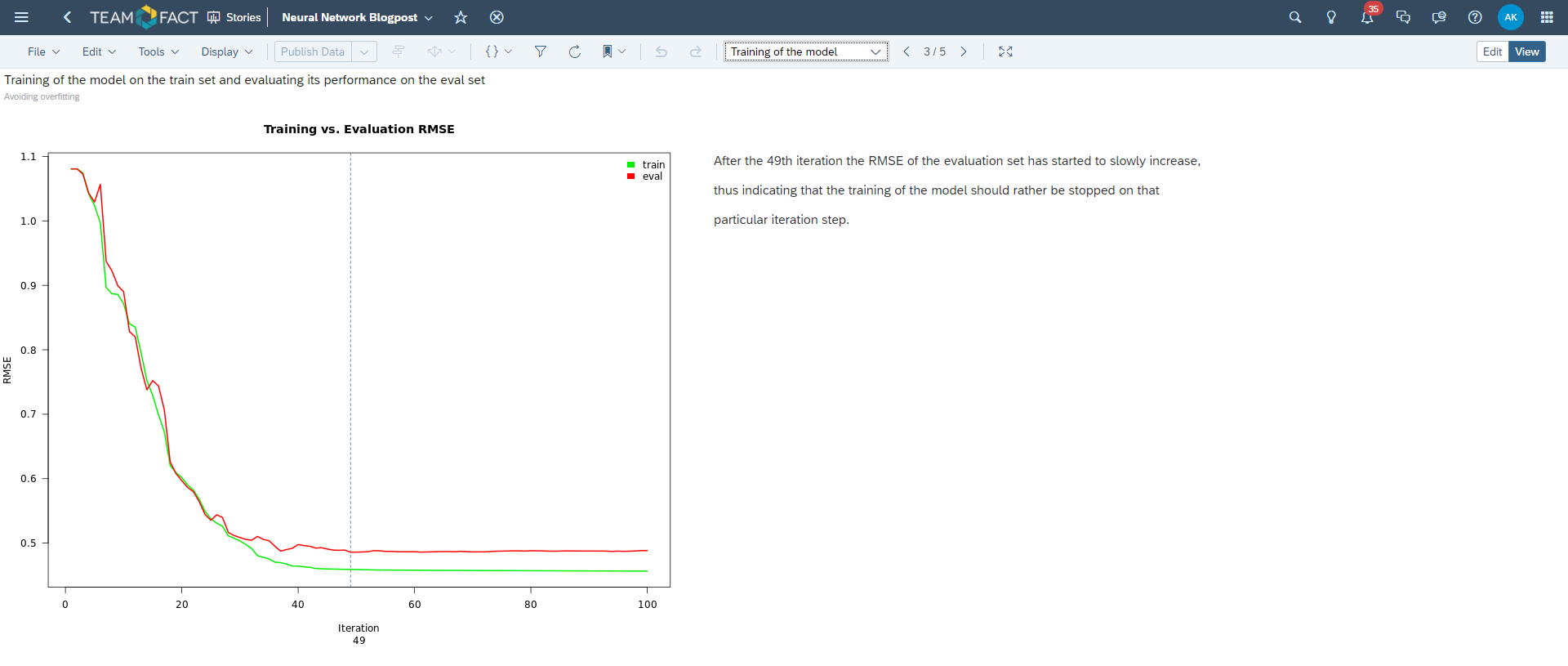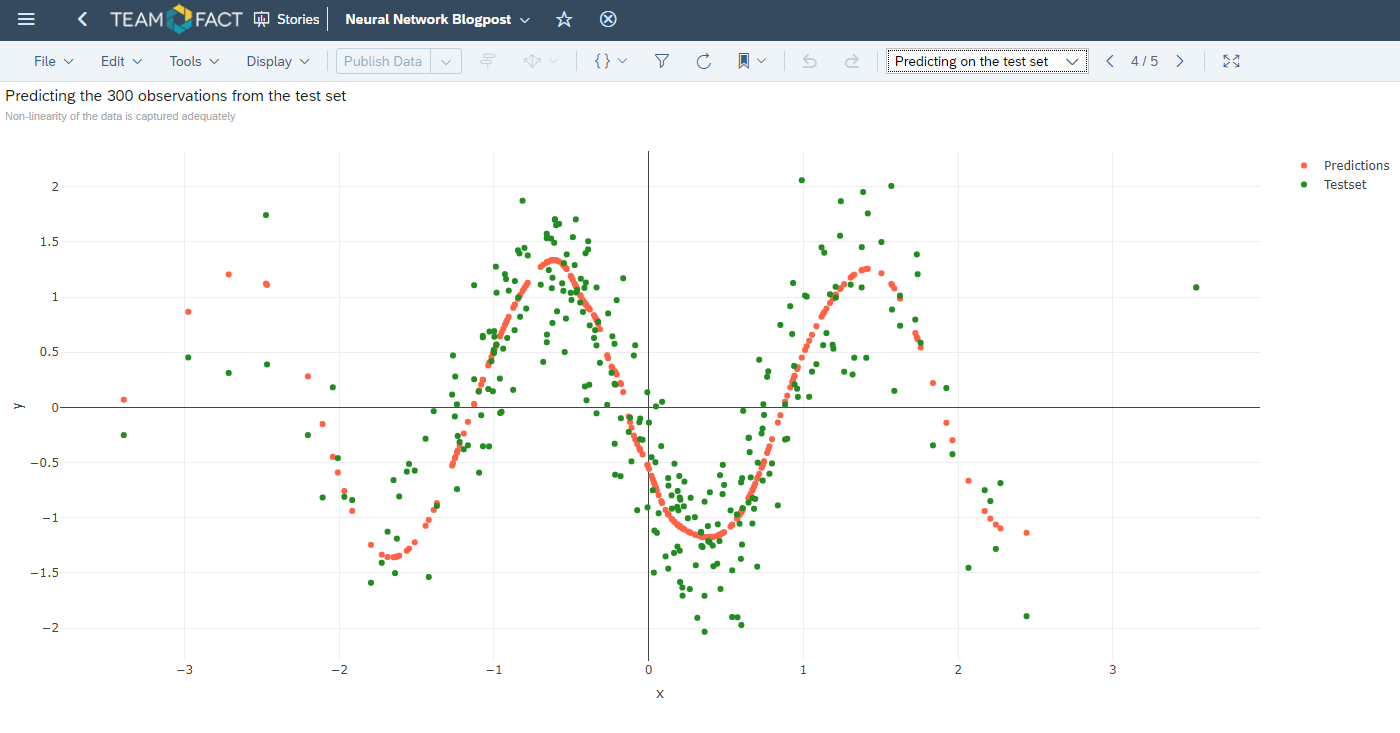# Introduction

Neural Networks are a powerful mechanism to model complex relationships between dependent variable and one or more independent variables.

The dependent variable can be either categorical or continuous and, thus, defines the prerequisites of the underlying modeling.

In case of a categorical dependent variable the error metric would be cross-entropy (binary or multinomial); in case of a continuous response - which is a regression problem as such - the error metric would be the standard RSS (residual sum of squares).

The goal is to minimize the loss function (the sum applied to the error metric entities) to achieve best fit possible on the training data, but not to overfit the model on this training data set and, thus, make robust and reliable predictions for the never seen before data feasible.

In this blogpost we will showcase the possibility of building a neural network model completely within the SAP Analytics Cloud in an R widget, which is available there for advanced R graphics and calculations.

No external additional software products will be used to achieve this goal.

### The Data we'll be modeling

Please first and foremost be aware that an R widget allows one to access any data available within SAC. This means that you can train a neural network using a data set or a data model already imported into the SAC environment previously.

For the purpose of this blogpost I'm using the data set showcasing a highly non-linear relationship between two variables (X and Y), as depicted in the screenshot below.

The dependent variable is the Y variable in this context (Y axis) and the X is the independent variable, which we will be using as a predictor in our model.

One can clearly observe the sheer necessity for non-linear modeling technique for these data, as i.e. a simple linear regression (straight line with an intercept and a slope) would definitely be inappropriate to model this kind of complexity:Please feel free to right click the image and open it separately in another window or tab to see a bigger picture... :-)

The code to generate this graphic in the R widget is listed below:

----

# 'dta' is a data set, which contains these data.

require(plotly)

fig <- plot_ly(dta, x = ~x, y = ~y, color = I("orange2"))

fig

----

In general this data set contains 2 000 observations of two variables (X and Y).

The next step would be to split it into training and test sets. The test set will not be used for modeling purpose at all, but only to assess the model's performance afterwards.

The training set will itself be splitted into the actual data, which will be used for the training of the neural network and the so called evaluation set, which is necessary to avoid overfitting of the underlying training set.The code to produce these two plots within two separate R widgets is listed below:

----

# Training data plot R widget graphic #

require(plotly)

set.seed(42)
test_ids <- sample(1 : nrow(dta), size = 300, replace = FALSE)
test_set <- dta[test_ids, ]
train_set <- dta[-test_ids, ]

x_mean <- mean(train_set\$x); x_sd <- sd(train_set\$x)
y_mean <- mean(train_set\$y); y_sd <- sd(train_set\$y)

train_set\$x <- (train_set\$x - x_mean) / x_sd
train_set\$y <- (train_set\$y - y_mean) / y_sd

fig <- plot_ly(train_set, x = ~x, y = ~y, color = I("orange2"))
fig

----

# Test data plot R widget graphic #

require(plotly)

set.seed(42)
test_ids <- sample(1 : nrow(dta), size = 300, replace = FALSE)
test_set <- dta[test_ids, ]
train_set <- dta[-test_ids, ]

x_mean <- mean(train_set\$x); x_sd <- sd(train_set\$x)
y_mean <- mean(train_set\$y); y_sd <- sd(train_set\$y)

test_set\$x <- (test_set\$x - x_mean) / x_sd
test_set\$y <- (test_set\$y - y_mean) / y_sd

fig <- plot_ly(test_set, x = ~x, y = ~y, color = I("forestgreen"))
fig

----

### Training of the neural network model

As of right now, we are ready to train the neural network model completely within the SAP Analytics Cloud infrastructure in an R widget framework.

For this purpose the evaluation data set has been derived from the training data and used to monitor the progress of the neural network training.

You can see the semantics of this evaluation set and the training progress loop in the code snippet below:

----

require(nnet)

set.seed(42)
test_ids <- sample(1 : nrow(dta), size = 300, replace = FALSE)
test_set <- dta[test_ids, ]
train_set <- dta[-test_ids, ]

x_mean <- mean(train_set\$x); x_sd <- sd(train_set\$x)
y_mean <- mean(train_set\$y); y_sd <- sd(train_set\$y)

train_set\$x <- (train_set\$x - x_mean) / x_sd
train_set\$y <- (train_set\$y - y_mean) / y_sd

test_set\$x <- (test_set\$x - x_mean) / x_sd
test_set\$y <- (test_set\$y - y_mean) / y_sd

set.seed(42)
eval_ids <- sample(1 : nrow(train_set), size = 500, replace = FALSE)
eval_set <- train_set[eval_ids, ]
train_set <- train_set[-eval_ids, ]

metrics <- data.frame()

for (i in 1 : 100) {
set.seed(1337)
nn <- nnet(y ~ x, data = train_set, size = 40, maxit = i, linout = TRUE, rang = 0.7)
RMSE_train <- sqrt(mean((train_set\$y - predict(nn))^2))
RMSE_eval  <- sqrt(mean((eval_set\$y  - predict(nn, newdata = eval_set))^2))
metrics <- rbind(metrics, data.frame(RMSE_train, RMSE_eval))
}

plot(1 : nrow(metrics), metrics\$RMSE_train, type = "l", col = "green2", lwd = 2,
main = "Training vs. Evaluation RMSE", xlab = "Iteration", ylab = "RMSE", las = 1)
lines(1 : nrow(metrics), metrics\$RMSE_eval, col = "red", lwd = 2, xpd = TRUE)
legend("topright", legend = c("train", "eval"), fill = c("green2", "red"), border = NA, bty = "n")
abline(v = which.min(metrics\$RMSE_eval), col = "steelblue4", lty = 2)
title(sub = which.min(metrics\$RMSE_eval))

----

The corresponding result of this process is depicted in the plot below. Please note that the 49th iteration of this fully connected feed forward neural network presents the minimum for the loss function on the evaluation data set and, thus, should be considered as a pivotal point of the training progress.

The training should be stopped at this particular iteration.

This technique is called cross-validation and serves as a means to avoid overfitting of the underlying training data set:I have only used one-fold cross-validation here for the sake of the simplicity, - it is, however, prudent to apply the so called n-fold cross-validation, where this process is repeated multiple times to ensure the stable local minimum of the optimization algorithm (the pivotal iteration should be roughly the same for all folds), and finally take an average or the median of the accrued iteration numbers for the final complete training of the neural network model.

If you still remember, we have splitted the initial data into the training and the test sets as the first preliminary step towards the actual training of the model.

Now, it's time to get back to this test set and feed it into the finally trained neural network to assess the performance of the elaborated model.

The code to achieve this looks the following:

----

require(nnet)
require(plotly)

set.seed(42)
test_ids <- sample(1 : nrow(dta), size = 300, replace = FALSE)
test_set <- dta[test_ids, ]
train_set <- dta[-test_ids, ]

x_mean <- mean(train_set\$x); x_sd <- sd(train_set\$x)
y_mean <- mean(train_set\$y); y_sd <- sd(train_set\$y)

train_set\$x <- (train_set\$x - x_mean) / x_sd
train_set\$y <- (train_set\$y - y_mean) / y_sd

test_set\$x <- (test_set\$x - x_mean) / x_sd
test_set\$y <- (test_set\$y - y_mean) / y_sd

set.seed(42)
eval_ids <- sample(1 : nrow(train_set), size = 500, replace = FALSE)
eval_set <- train_set[eval_ids, ]
train_set <- train_set[-eval_ids, ]

set.seed(1337)
nn <- nnet(y ~ x, data = rbind(train_set, eval_set), size = 40, maxit = 49, linout = TRUE, rang = 0.7)

pred <- rbind(test_set[, -1], data.frame("x" = test_set\$x, "y" = predict(nn, newdata = test_set)))
pred\$color <- c(rep("Testset", 300), rep("Predictions", 300))
pred\$color <- as.factor(pred\$color)

fig <- plot_ly(pred, x = ~x, y = ~y, color = ~color, colors = c("tomato", "forestgreen"))
fig

----

And the corresponding graphical output is shown below:One can clearly see that the severe non-linearity has been captured adequately by the neural network model and the used cross-validation did indeed prohibit the model to be overfitted on the training data set, thus giving a robust and obviously very coherent and adequate predictions for these never seen before data set observations.

As the last touch, I'm willing to depict the neural network topology used to model these data. It can clearly be derived from the source code, I've already presented above, but, nevertheless, the graphical representation is always more obvious and, thus, quite welcome:### Final thoughts

Neural Networks are not new on the field of statistical modeling and research. They have been around for already quite some time now.

Despite their power in predicting the data, they are quite cumbersome to deal with when it comes to explaining the relationship(s) between the outcome and the predictor(s).

Please use this technique with utmost care and do not exaggerate its usefulness.

Sometimes it's better and more versatile to use a simpler modeling approach if no complex structure is observed in the data to be modeled.

However, if the underlying relationship's behaviour between the outcome and the predictors is prone to the highly non-linear pattern structures, - the neural network can potentially be a plausible solution for the modeling.

Please be sure to use the cross-validation technique to avoid overfitting of the actual training data.

All things considered: Have fun while modeling!